# 写给程序员的机器学习入门 (三) - 线性模型，激活函数与多层线性模型

+关注继续查看

（因为我不是专家，这里的解释只用于理解人工神经元模拟了生物神经元的什么地方，不一定完全准确）

h11 = g(x1 w111 + x2 w112 + x3 * w113 + b11)
h12 = g(x1 w121 + x2 w122 + x3 * w123 + b12)
h21 = g(h11 w211 + h12 w212 + b21)
h22 = g(h11 w221 + h12 w222 + b22)
y = g(h21 w311 + h22 w312 + b31)

import torch

# 创建 pytorch 封装的线性模型，设置输入有 3 个输出有 1 个

model = torch.nn.Linear(in_features=3, out_features=1)

# 初始值会随机生成 (使用 kaiming_uniform 生成正态分布)

list(model.parameters())

[Parameter containing:
tensor([[0.0599, 0.1324, 0.0099]], requires_grad=True), Parameter containing:

# 定义输入和输出

x = torch.tensor([1, 2, 3], dtype=torch.float)
y = torch.tensor(, dtype=torch.float)

p = model(x)

p

# 计算误差并自动微分

l = (p - y).abs()
l

l.backward()

# 查看各个参数对应的导函数值

tensor([[-1., -2., -3.]])

tensor([-1.])

import torch

# 创建 pytorch 封装的线性模型，设置输入有 3 个输出有 2 个

model = torch.nn.Linear(in_features=3, out_features=2)

# 第二个参数表示 2 个输出对应的 b 值 (偏移)

list(model.parameters())

[Parameter containing:
tensor([[0.1393, 0.5165, 0.2910],

    [0.2276, 0.1579, 0.1958]], requires_grad=True), Parameter containing:

# 定义输入和输出

x = torch.tensor([1, 2, 3], dtype=torch.float)
y = torch.tensor([6, -6], dtype=torch.float)

p = model(x)

p

# (abs(2.3019 - 6) + abs(1.3009 - -6)) / 2 = 5.4995

l = (p - y).abs().mean()
l

l.backward()

# 因为误差取了 2 个值的平均，所以求导函数值的时候会除以 2

tensor([[-0.5000, -1.0000, -1.5000],

    [ 0.5000,  1.0000,  1.5000]])

tensor([-0.5000, 0.5000])

import torch

# 这是为了让训练过程可重现，你也可以选择不这样做

torch.random.manual_seed(0)

# 创建线性模型，设置有 3 个输入 1 个输出

model = torch.nn.Linear(in_features=3, out_features=1)

# 创建损失计算器

loss_function = torch.nn.MSELoss()

# 创建参数调整器

optimizer = torch.optim.SGD(model.parameters(), lr=0.01)

# 随机生成原始数据集，一共 20 组数据，每条数据有 3 个输入

dataset_x = torch.randn((20, 3))
dataset_y = dataset_x.mm(torch.tensor([, , ], dtype=torch.float)) + 8
print(f"dataset_x: {dataset_x}")
print(f"dataset_y: {dataset_y}")

# 切分训练集 (12 组)，验证集 (4 组) 和测试集 (4 组)

random_indices = torch.randperm(dataset_x.shape)
traning_indices = random_indices[:int(len(random_indices)*0.6)]
validating_indices = random_indices[int(len(random_indices)0.6):int(len(random_indices)0.8):]
testing_indices = random_indices[int(len(random_indices)*0.8):]
traning_set_x = dataset_x[traning_indices]
traning_set_y = dataset_y[traning_indices]
validating_set_x = dataset_x[validating_indices]
validating_set_y = dataset_y[validating_indices]
testing_set_x = dataset_x[testing_indices]
testing_set_y = dataset_y[testing_indices]

# 开始训练过程

for epoch in range(1, 10000):

print(f"epoch: {epoch}")

# 根据训练集训练并修改参数
# 切换模型到训练模式，将会启用自动微分，批次正规化 (BatchNorm) 与 Dropout
model.train()

# 计算预测值
# 20 行 3 列的矩阵乘以 3 行 1 列的矩阵 (由 weight 转置得到) 等于 20 行 1 列的矩阵
predicted = model(traning_set_x)
# 计算损失
loss = loss_function(predicted, traning_set_y)
# 打印除错信息
print(f"loss: {loss}, weight: {model.weight}, bias: {model.bias}")
# 从损失自动微分求导函数值
loss.backward()
# 使用参数调整器调整参数
optimizer.step()
# 清空导函数值

# 检查验证集
# 切换模型到验证模式，将会禁用自动微分，批次正规化 (BatchNorm) 与 Dropout
model.eval()
predicted = model(validating_set_x)
validating_accuracy = 1 - ((validating_set_y - predicted).abs() / validating_set_y).abs().mean()
print(f"validating x: {validating_set_x}, y: {validating_set_y}, predicted: {predicted}")

# 如果验证集正确率大于 99 %，则停止训练
print(f"validating accuracy: {validating_accuracy}")
if validating_accuracy > 0.99:
break


# 检查测试集

predicted = model(testing_set_x)
testing_accuracy = 1 - ((testing_set_y - predicted).abs() / testing_set_y).abs().mean()
print(f"testing x: {testing_set_x}, y: {testing_set_y}, predicted: {predicted}")
print(f"testing accuracy: {testing_accuracy}")

dataset_x: tensor([[ 0.8487, 0.6920, -0.3160],

    [-2.1152, -0.3561,  0.4372],
[ 0.4913, -0.2041,  0.1198],
[ 1.2377,  1.1168, -0.2473],
[-1.0438, -1.3453,  0.7854],
[ 0.9928,  0.5988, -1.5551],
[-0.3414,  1.8530,  0.4681],
[-0.1577,  1.4437,  0.2660],
[ 1.3894,  1.5863,  0.9463],
[-0.8437,  0.9318,  1.2590],
[ 2.0050,  0.0537,  0.4397],
[ 0.1124,  0.6408,  0.4412],
[-0.2159, -0.7425,  0.5627],
[ 0.2596,  0.5229,  2.3022],
[-1.4689, -1.5867, -0.5692],
[ 0.9200,  1.1108,  1.2899],
[-1.4782,  2.5672, -0.4731],
[ 0.3356, -1.6293, -0.5497],
[-0.4798, -0.4997, -1.0670],
[ 1.1149, -0.1407,  0.8058]])

dataset_y: tensor([[ 9.2847],

    [ 6.4842],
[ 8.4426],
[10.7294],
[ 6.6217],
[ 5.5252],
[12.7689],
[11.5278],
[15.4009],
[12.7970],
[11.4315],
[10.7175],
[ 7.9872],
[16.2120],
[ 1.6500],
[15.0112],
[10.2369],
[ 3.4277],
[ 3.3199],
[11.2509]])

epoch: 1
loss: 142.77590942382812, weight: Parameter containing:
tensor([[-0.0043, 0.3097, -0.4752]], requires_grad=True), bias: Parameter containing:
validating x: tensor([[-0.4798, -0.4997, -1.0670],

    [ 0.8487,  0.6920, -0.3160],
[ 0.1124,  0.6408,  0.4412],
[-1.0438, -1.3453,  0.7854]]), y: tensor([[ 3.3199],
[ 9.2847],
[10.7175],
[ 6.6217]]), predicted: tensor([[-0.1385],
[ 0.3020],
[-0.0126],
[-1.1801]], grad_fn=<AddmmBackward>)

validating accuracy: -0.04714548587799072
epoch: 2
loss: 131.40403747558594, weight: Parameter containing:
tensor([[ 0.0675, 0.4937, -0.3163]], requires_grad=True), bias: Parameter containing:
validating x: tensor([[-0.4798, -0.4997, -1.0670],

    [ 0.8487,  0.6920, -0.3160],
[ 0.1124,  0.6408,  0.4412],
[-1.0438, -1.3453,  0.7854]]), y: tensor([[ 3.3199],
[ 9.2847],
[10.7175],
[ 6.6217]]), predicted: tensor([[-0.2023],
[ 0.6518],
[ 0.3935],
[-1.1479]], grad_fn=<AddmmBackward>)

validating accuracy: -0.03184401988983154
epoch: 3
loss: 120.98343658447266, weight: Parameter containing:
tensor([[ 0.1357, 0.6687, -0.1639]], requires_grad=True), bias: Parameter containing:
validating x: tensor([[-0.4798, -0.4997, -1.0670],

    [ 0.8487,  0.6920, -0.3160],
[ 0.1124,  0.6408,  0.4412],
[-1.0438, -1.3453,  0.7854]]), y: tensor([[ 3.3199],
[ 9.2847],
[10.7175],
[ 6.6217]]), predicted: tensor([[-0.2622],
[ 0.9860],
[ 0.7824],
[-1.1138]], grad_fn=<AddmmBackward>)

validating accuracy: -0.016991496086120605

epoch: 637
loss: 0.001102567883208394, weight: Parameter containing:
tensor([[1.0044, 2.0283, 3.0183]], requires_grad=True), bias: Parameter containing:
validating x: tensor([[-0.4798, -0.4997, -1.0670],

    [ 0.8487,  0.6920, -0.3160],
[ 0.1124,  0.6408,  0.4412],
[-1.0438, -1.3453,  0.7854]]), y: tensor([[ 3.3199],
[ 9.2847],
[10.7175],
[ 6.6217]]), predicted: tensor([[ 3.2395],
[ 9.2574],
[10.6993],
[ 6.5488]], grad_fn=<AddmmBackward>)

validating accuracy: 0.9900396466255188
testing x: tensor([[-0.3414, 1.8530, 0.4681],

    [-1.4689, -1.5867, -0.5692],
[ 1.1149, -0.1407,  0.8058],
[ 0.3356, -1.6293, -0.5497]]), y: tensor([[12.7689],
[ 1.6500],
[11.2509],
[ 3.4277]]), predicted: tensor([[12.7834],
[ 1.5438],
[11.2217],
[ 3.3285]], grad_fn=<AddmmBackward>)

testing accuracy: 0.9757462739944458

def relu(x):

if x > 0:
return x
return 0

def relu(x):

if x > 0:
return x
return 0


for x in range(1, 11):

h1 = relu(x * -1 + 2)
h2 = relu(x * -1 + 3)
h3 = relu(x * -1 + 4)
y = h1 * 10 + h2 * 2 + h3 * 3 + 7
print(x, y)

1 30
2 15
3 10
4 7
5 7
6 7
7 7
8 7
9 7
10 7

https://pytorch.org/docs/stable/nn.html#non-linear-activations-weighted-sum-nonlinearity

x = torch.tensor(2.0)
y = torch.nn.functional.relu(x w1 + b1) w2 + b2

# 假设我们要调整 w1, b1, w2, b2 使得 y 接近 0

y

y.abs().backward()

tensor(12.)

tensor(6.)

tensor(11.)

# b1 的导函数值为 1

tensor(1.)

x = torch.tensor(2.0)
y = torch.nn.functional.relu(x w1 + b1) w2 + b2

y

y.backward()

tensor(-0.)

tensor(0.)

tensor(0.)

tensor(1.)

Sigmoid 和 Tanh 虽然有梯度消失问题，但是它们可以用于在指定场景下转换数值到 0 ~ 1 和 -1 ~ 1。例如 Sigmoid 可以用在最后一层表现可能性，100 表示非常有可能 (转换到 1)，50 也代表非常有可能 (转换到 1)，1 代表比较有可能 (转换到 0.7311)，0 代表不确定 (转换到 0.5)，-1 代表比较不可能 (转换到 0.2689)，-100 代表很不可能 (转换到 0)。而后面文章介绍的 LSTM 模型也会使用 Sigmoid 决定需要忘记哪些内部状态，Tanh 决定应该怎样更新内部状态。此外还有 Softmax 等一般只用在最后一层的函数，Softmax 可以用于在分类的时候判断哪个类别可能性最大，例如识别猫狗猪的时候最后一层给出 6, 5, 8，数值越大代表属于该分类的可能性越高，经过 Softmax 转换以后就是 0.1142, 0.0420, 0.8438，代表有 11.42% 的可能性是猫，4.2% 的可能性是狗，84.38% 的可能性是猪。

# 引用 pytorch，nn 等同于 torch.nn

import torch
from torch import nn

# 定义模型

class MyModel(nn.Module):

def __init__(self):
# 初始化基类
super().__init__()
# 定义参数
# 这里一共定义了三层
#   第一层接收 2 个输入，返回 32 个隐藏值 (内部 weight 矩阵为 32 行 2 列)
#   第二层接收 32 个隐藏值，返回 64 个隐藏值 (内部 weight 矩阵为 64 行 32 列)
#   第三层接收 64 个隐藏值，返回 1 个输出 (内部 weight 矩阵为 1 行 64 列)
self.layer1 = nn.Linear(in_features=2, out_features=32)
self.layer2 = nn.Linear(in_features=32, out_features=64)
self.layer3 = nn.Linear(in_features=64, out_features=1)

def forward(self, x):
# x 是一个矩阵，行数代表批次，列数代表输入个数，例如有 50 个批次则为 50 行 2 列

# 计算第一层返回的隐藏值，例如有 50 个批次则 hidden1 为 50 行 32 列
# 计算矩阵乘法时会转置 layer1 内部的 weight 矩阵，50 行 2 列乘以 2 行 32 列等于 50 行 32 列
hidden1 = nn.functional.relu(self.layer1(x))

# 计算第二层返回的隐藏值，例如有 50 个批次则 hidden2 为 50 行 64 列
hidden2 = nn.functional.relu(self.layer2(hidden1))

# 计算第三层返回的输出，例如有 50 个批次则 y 为 50 行 1 列
y = self.layer3(hidden2)

# 返回输出
return y


# 创建模型实例

model = MyModel()

# 引用 pytorch，nn 等同于 torch.nn

import torch
from torch import nn

# 创建模型实例，效果等同于第一种方法

model = nn.Sequential(

nn.Linear(in_features=2, out_features=32),
nn.ReLU(),
nn.Linear(in_features=32, out_features=64),
nn.ReLU(),
nn.Linear(in_features=64, out_features=1))


# nn.functional.relu(x) 等于 nn.ReLU()(x)

29,0,0,1,2,2,1,4,12500
22,0,2,2,3,1,2,5,15500
24,0,4,1,2,1,1,2,16000
35,0,6,3,3,0,1,0,19500
45,0,18,0,5,2,0,5,17000
24,0,2,0,0,0,1,1,13500
23,1,2,2,3,1,1,0,10500
41,0,16,2,5,5,2,0,16500
50,0,18,0,5,0,5,2,16500
20,0,0,0,5,2,0,1,12500
26,0,6,1,5,5,1,1,27000
46,0,12,0,5,4,4,2,12500
26,0,6,1,5,3,1,1,23500
40,0,9,0,0,1,0,1,17500
41,0,20,3,5,3,3,5,20500
26,0,4,0,1,2,4,0,18500
42,0,18,5,0,0,2,5,18500
21,0,1,1,0,1,2,0,12000
26,0,1,0,0,0,0,2,12500

Java 编码熟练程度 (0 ~ 5)
NET 编码熟练程度 (0 ~ 5)
JS 编码熟练程度 (0 ~ 5)
CSS 编码熟练程度 (0 ~ 5)
HTML 编码熟练程度 (0 ~ 5)

# 引用 pytorch 和 pandas

import pandas
import torch
from torch import nn

# 定义模型

class MyModel(nn.Module):

def __init__(self):
super().__init__()
self.layer1 = nn.Linear(in_features=8, out_features=100)
self.layer2 = nn.Linear(in_features=100, out_features=50)
self.layer3 = nn.Linear(in_features=50, out_features=1)

def forward(self, x):
hidden1 = nn.functional.relu(self.layer1(x))
hidden2 = nn.functional.relu(self.layer2(hidden1))
y = self.layer3(hidden2)
return y


# 这是为了让训练过程可重现，你也可以选择不这样做

torch.random.manual_seed(0)

# 创建模型实例

model = MyModel()

# 创建损失计算器

loss_function = torch.nn.MSELoss()

# 创建参数调整器

optimizer = torch.optim.SGD(model.parameters(), lr=0.0000001)

# 从 csv 读取原始数据集

dataset_tensor = torch.tensor(df.values, dtype=torch.float)

# 切分训练集 (60%)，验证集 (20%) 和测试集 (20%)

random_indices = torch.randperm(dataset_tensor.shape)
traning_indices = random_indices[:int(len(random_indices)*0.6)]
validating_indices = random_indices[int(len(random_indices)0.6):int(len(random_indices)0.8):]
testing_indices = random_indices[int(len(random_indices)*0.8):]
traning_set_x = dataset_tensortraning_indices
traning_set_y = dataset_tensortraning_indices
validating_set_x = dataset_tensorvalidating_indices
validating_set_y = dataset_tensorvalidating_indices
testing_set_x = dataset_tensortesting_indices
testing_set_y = dataset_tensortesting_indices

# 开始训练过程

for epoch in range(1, 1000):

print(f"epoch: {epoch}")

# 根据训练集训练并修改参数
# 切换模型到训练模式，将会启用自动微分，批次正规化 (BatchNorm) 与 Dropout
model.train()

for batch in range(0, traning_set_x.shape, 100):
# 切分批次，一次只计算 100 组数据
batch_x = traning_set_x[batch:batch+100]
batch_y = traning_set_y[batch:batch+100]
# 计算预测值
predicted = model(batch_x)
# 计算损失
loss = loss_function(predicted, batch_y)
# 从损失自动微分求导函数值
loss.backward()
# 使用参数调整器调整参数
optimizer.step()
# 清空导函数值

# 检查验证集
# 切换模型到验证模式，将会禁用自动微分，批次正规化 (BatchNorm) 与 Dropout
model.eval()
predicted = model(validating_set_x)
validating_accuracy = 1 - ((validating_set_y - predicted).abs() / validating_set_y).mean()
print(f"validating x: {validating_set_x}, y: {validating_set_y}, predicted: {predicted}")
print(f"validating accuracy: {validating_accuracy}")


# 检查测试集

predicted = model(testing_set_x)
testing_accuracy = 1 - ((testing_set_y - predicted).abs() / testing_set_y).mean()
print(f"testing x: {testing_set_x}, y: {testing_set_y}, predicted: {predicted}")
print(f"testing accuracy: {testing_accuracy}")

# 手动输入数据预测输出

while True:

try:
print("enter input:")
r = list(map(float, input().split(",")))
x = torch.tensor(r).view(1, len(r))
print(model(x)[0,0].item())
except Exception as e:
print("error:", e)

epoch: 1
validating x: tensor([[42., 0., 16., ..., 5., 5., 5.],

    [28.,  0.,  0.,  ...,  4.,  0.,  0.],
[23.,  0.,  3.,  ...,  0.,  1.,  1.],
...,
[44.,  1., 15.,  ...,  2.,  0.,  2.],
[30.,  0.,  1.,  ...,  1.,  1.,  2.],
[50.,  1., 18.,  ...,  5.,  5.,  2.]]), y: tensor([[24500.],
[12500.],
[17500.],
...,
[10500.],
[15000.],
[16000.]]), predicted: tensor([[27604.2578],
[15934.7607],
[14536.8984],
...,
[23678.5547],
[18189.6953],
[29968.8789]], grad_fn=<AddmmBackward>)

validating accuracy: 0.661293625831604
epoch: 2
validating x: tensor([[42., 0., 16., ..., 5., 5., 5.],

    [28.,  0.,  0.,  ...,  4.,  0.,  0.],
[23.,  0.,  3.,  ...,  0.,  1.,  1.],
...,
[44.,  1., 15.,  ...,  2.,  0.,  2.],
[30.,  0.,  1.,  ...,  1.,  1.,  2.],
[50.,  1., 18.,  ...,  5.,  5.,  2.]]), y: tensor([[24500.],
[12500.],
[17500.],
...,
[10500.],
[15000.],
[16000.]]), predicted: tensor([[29718.2441],
[15790.3799],
[15312.5791],
...,
[23395.9668],
[18672.0234],
[31012.4062]], grad_fn=<AddmmBackward>)

validating accuracy: 0.6694601774215698

epoch: 999
validating x: tensor([[42., 0., 16., ..., 5., 5., 5.],

    [28.,  0.,  0.,  ...,  4.,  0.,  0.],
[23.,  0.,  3.,  ...,  0.,  1.,  1.],
...,
[44.,  1., 15.,  ...,  2.,  0.,  2.],
[30.,  0.,  1.,  ...,  1.,  1.,  2.],
[50.,  1., 18.,  ...,  5.,  5.,  2.]]), y: tensor([[24500.],
[12500.],
[17500.],
...,
[10500.],
[15000.],
[16000.]]), predicted: tensor([[22978.7656],
[13050.8018],
[18396.5176],
...,
[11449.5059],
[14791.2969],
[16635.2578]], grad_fn=<AddmmBackward>)

validating accuracy: 0.9311849474906921
testing x: tensor([[48., 1., 18., ..., 5., 0., 5.],

    [22.,  1.,  2.,  ...,  2.,  1.,  2.],
[24.,  0.,  1.,  ...,  3.,  2.,  0.],
...,
[24.,  0.,  4.,  ...,  0.,  1.,  1.],
[39.,  0.,  0.,  ...,  0.,  5.,  5.],
[36.,  0.,  5.,  ...,  3.,  0.,  3.]]), y: tensor([[14000.],
[10500.],
[13000.],
...,
[15500.],
[12000.],
[19000.]]), predicted: tensor([[15481.9062],
[11011.7266],
[12192.7949],
...,
[16219.3027],
[11074.0420],
[20305.3516]], grad_fn=<AddmmBackward>)

testing accuracy: 0.9330180883407593
enter input:

enter input:
35,0,10,5,2,1,1,2
26790.982421875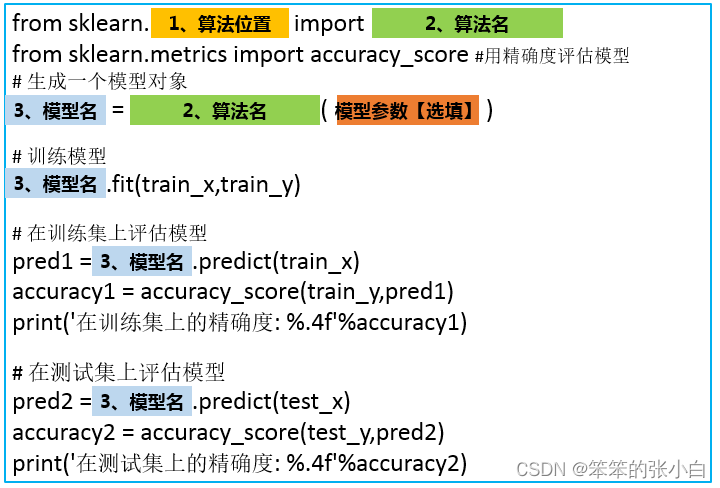0 00 0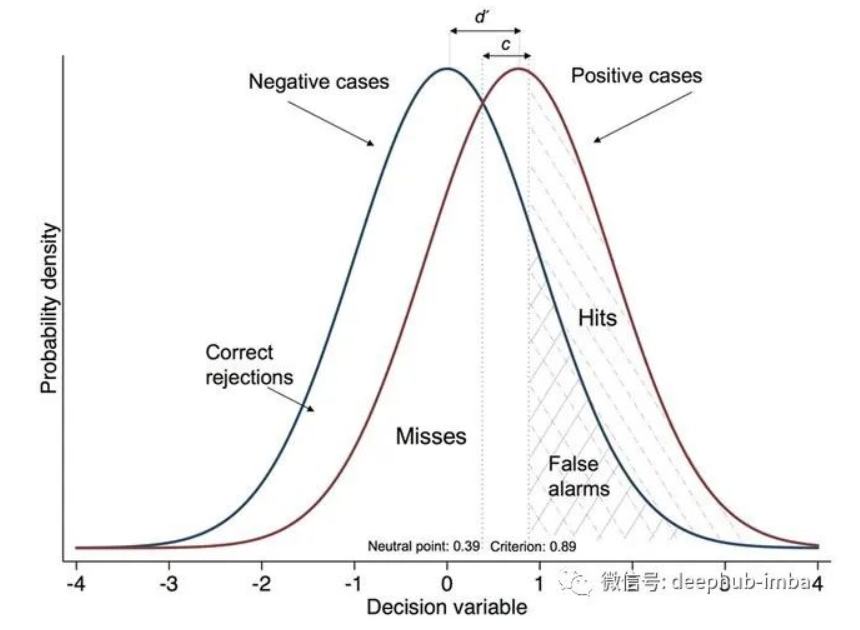0 0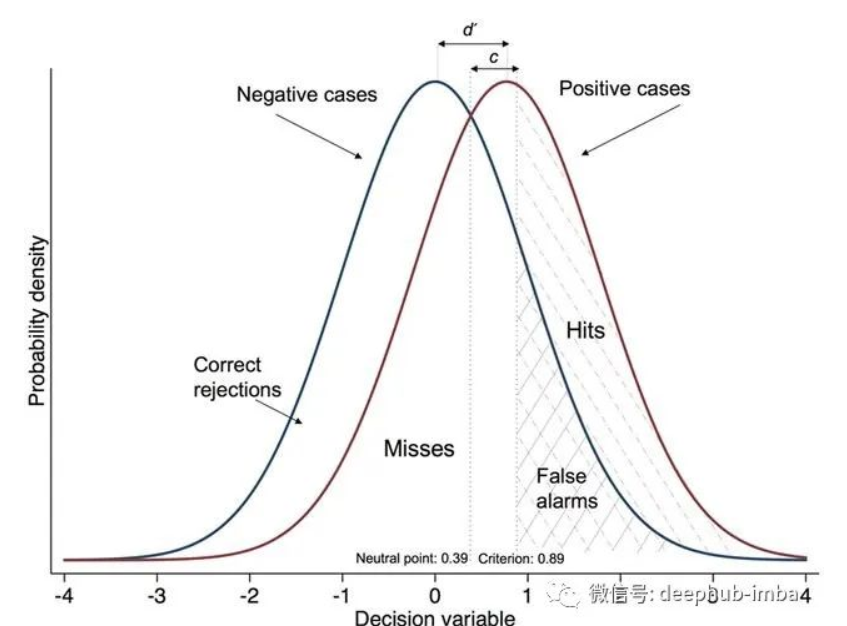0 0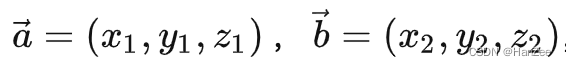0 0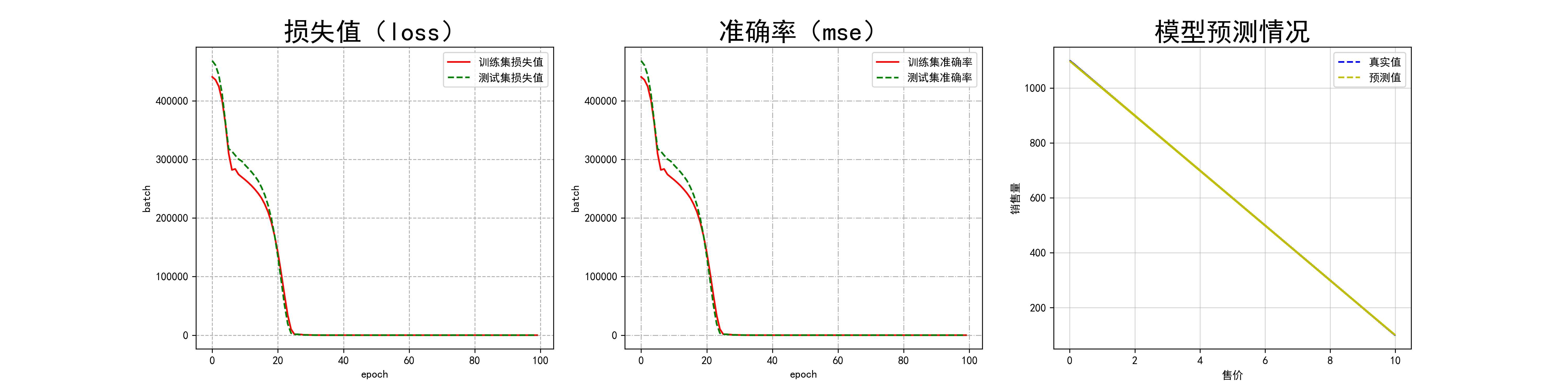【人工智能】机器学习入门之监督学习（一）有监督学习
【人工智能】机器学习入门之监督学习（一）有监督学习
0 0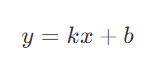0 0R 语言是为数学研究工作者设计的一种数学编程语言，主要用于统计分析、绘图、数据挖掘。 机器学习是一门多领域交叉学科，涉及概率论、统计学、逼近论、凸分析、算法复杂度理论等多门学科。专门研究计算机怎样模拟或实现人类的学习行为，以获取新的知识或技能，重新组织已有的知识结构使之不断改善自身的性能。
0 0

【算法实战】9. 线性回归算法275

【算法实战】5. Logistic回归算法376

【算法实战】4. 朴素贝叶斯算法976

【算法实战】6. 支持向量机算法4910517

【算法实战】2. K近邻算法1255